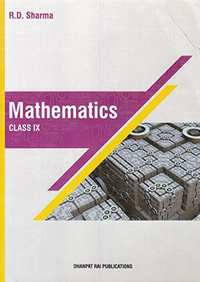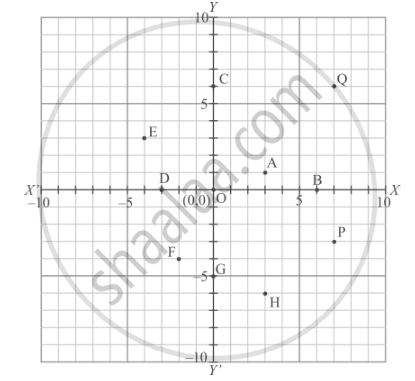# RD Sharma solutions for Mathematics for Class 9 chapter 8 - Co-ordinate Geometry [Latest edition]

#### Chapters## Chapter 8: Co-ordinate Geometry

Exercise 8.1Exercise 8.2
Exercise 8.1 [Page 6]

### RD Sharma solutions for Mathematics for Class 9 Chapter 8 Co-ordinate Geometry Exercise 8.1 [Page 6]

Exercise 8.1 | Q 1.01 | Page 6

Plot the following point on the graph paper:

(2,5)

Exercise 8.1 | Q 1.02 | Page 6

Plot the following point on the graph paper:

(4, −3)

Exercise 8.1 | Q 1.03 | Page 6

Plot the following point on the graph paper:

(−5, −7)

Exercise 8.1 | Q 1.04 | Page 6

Plot the following point on the graph paper:

(7, −4)

Exercise 8.1 | Q 1.05 | Page 6

Plot the following point on the graph paper:

(−3, 2)

Exercise 8.1 | Q 1.06 | Page 6

Plot the following point on the graph paper:

(7, 0)

Exercise 8.1 | Q 1.07 | Page 6

Plot the following point on the graph paper:

(−4, 0)

Exercise 8.1 | Q 1.08 | Page 6

Plot the following point on the graph paper:

(0, 7)

Exercise 8.1 | Q 1.09 | Page 6

Plot the following point on the graph paper:

(0, −4)

Exercise 8.1 | Q 1.1 | Page 6

Plot the following point on the graph paper:

(0, 0)

Exercise 8.1 | Q 2 | Page 6

Write the coordinates of each of the following points marked in the graph paper:Exercise 8.2 [Page 7]

### RD Sharma solutions for Mathematics for Class 9 Chapter 8 Co-ordinate Geometry Exercise 8.2 [Page 7]

Exercise 8.2 | Q 1 | Page 7

Mark the correct alternative in each of the following:
The point of intersect of the coordinate axes is

• ordinate

• abscissa

•  origin

Exercise 8.2 | Q 2 | Page 7

The abscissa and ordinate of the origin are

• (0, 0)

• (1, 0)

• (0, 1)

• (1 , 1)

Exercise 8.2 | Q 3 | Page 7

The measure of the angle between the coordinate axes is

• 90°

• 180°

• 360°

Exercise 8.2 | Q 4 | Page 7

A point whose abscissa and ordinate are 2 and −5 respectively, lies in

Exercise 8.2 | Q 5 | Page 7

Points (−4, 0) and (7, 0) lie

• on x-axis

• y-axis

Exercise 8.2 | Q 6 | Page 7

The ordinate of any point on x-axis is

• 0

• 1

• -1

• any number

Exercise 8.2 | Q 7 | Page 7

The abscissa of any point on y-axis is

• 0

• 1

• -1

• any number

Exercise 8.2 | Q 8 | Page 7

The abscissa of a point is positive in the

Exercise 8.2 | Q 9 | Page 7

A point whose abscissa is −3 and ordinate 2 lies in

Exercise 8.2 | Q 10 | Page 7

Two points having same abscissae but different ordinate lie on

• x-axis

• y-axis

• a line parallel to y-axis

• a line parallel to x-axis

Exercise 8.2 | Q 11 | Page 7

The perpendicular distance of the point P (4, 3) from x-axis is

• 4

• 3

• 5

• none of these

Exercise 8.2 | Q 12 | Page 7

The perpendicular distance of the P (4,3)  from y-axis is

• 4

• 3

• 5

• none of these

Exercise 8.2 | Q 13 | Page 7

The distance of the point P (4, 3) from the origin is

• 4

• 3

• 5

• 7

Exercise 8.2 | Q 14 | Page 7

The area of the triangle formed by the points A(2,0) B(6,0)  and C(4,6) is

•  24 sq. units

• 12 sq. units

• 10 sq. units

• none of these

Exercise 8.2 | Q 15 | Page 7

The area of the triangle formed by the points P (0, 1), Q (0, 5) and R (3, 4) is

• 16 sq. units

• 8 sq. units

• 4 sq. units

• 6 sq. units

## Chapter 8: Co-ordinate Geometry

Exercise 8.1Exercise 8.2## RD Sharma solutions for Mathematics for Class 9 chapter 8 - Co-ordinate Geometry

RD Sharma solutions for Mathematics for Class 9 chapter 8 (Co-ordinate Geometry) include all questions with solution and detail explanation. This will clear students doubts about any question and improve application skills while preparing for board exams. The detailed, step-by-step solutions will help you understand the concepts better and clear your confusions, if any. Shaalaa.com has the CBSE Mathematics for Class 9 solutions in a manner that help students grasp basic concepts better and faster.

Further, we at Shaalaa.com provide such solutions so that students can prepare for written exams. RD Sharma textbook solutions can be a core help for self-study and acts as a perfect self-help guidance for students.

Concepts covered in Mathematics for Class 9 chapter 8 Co-ordinate Geometry are Cartesian Coordinate System, Plotting a Point in the Plane If Its Coordinates Are Given., Coordinate Geometry.

Using RD Sharma Class 9 solutions Co-ordinate Geometry exercise by students are an easy way to prepare for the exams, as they involve solutions arranged chapter-wise also page wise. The questions involved in RD Sharma Solutions are important questions that can be asked in the final exam. Maximum students of CBSE Class 9 prefer RD Sharma Textbook Solutions to score more in exam.

Get the free view of chapter 8 Co-ordinate Geometry Class 9 extra questions for Mathematics for Class 9 and can use Shaalaa.com to keep it handy for your exam preparation